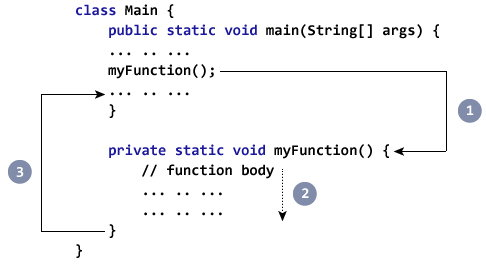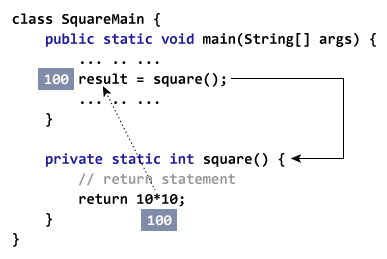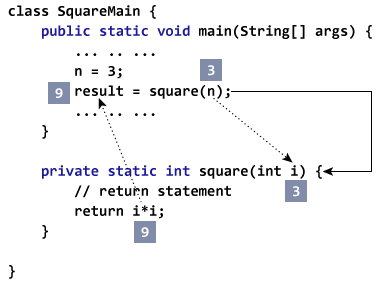# Java Methods

In this article, you’ll learn about Java methods, how to define a Java method and use them in your program with the help of examples.

## What is a method?

In mathematics, you might have studied about functions. For example, `f(x) = x2` is a function that returns squared value of x.

```If x = 2, then f(2) = 4
If x = 3, f(3) = 9
and so on.```

Similarly, in programming, a function is a block of code that performs a specific task.

In object-oriented programming, method is a jargon used for function. Methods are bound to a class and they define the behavior of a class.

Recommended Reading: Java Class and Objects

### Types of Java methods

Depending on whether a method is defined by the user, or available in standard library, there are two types of methods:

• Standard Library Methods
• User-defined Methods

### Standard Library Methods

The standard library methods are built-in methods in Java that are readily available for use. These standard libraries come along with the Java Class Library (JCL) in a Java archive (*.jar) file with JVM and JRE.

For example,

• `print()` is a method of `java.io.PrintSteam`. The `print("...")` prints the string inside quotation marks.
• `sqrt()` is a method of Math class. It returns square root of a number.

Here's an working example:

``````public class Numbers {
public static void main(String[] args) {
System.out.print("Square root of 4 is: " + Math.sqrt(4));
}
}``````

When you run the program, the output will be:

`Square root of 4 is: 2.0`

## User-defined Method

You can also define methods inside a class as per your wish. Such methods are called user-defined methods.

### How to create a user-defined method?

Before you can use (call a method), you need to define it.

Here is how you define methods in Java.

```public static void myMethod() {
System.out.println(“My Function called”);
}
```

Here, a method named `myMethod()` is defined.

You can see three keywords `public`, `static` and `void` before the function name.

• The `public` keyword makes `myMethod()` method `public`. Public members can be accessed from outside of the class. To learn more, visit: Java public and private Modifiers.
• The `static` keyword denotes that the method can be accessed without creating the object of the class. To learn more, visit: Static Keyword in Java
• The `void` keyword signifies that the method doesn’t return any value. You will learn about returning value from the method later in this article.

In the above program, our method doesn’t accept any arguments. Hence the empty parenthesis (). You will learn about passing arguments to a method later in this article.

The complete syntax for defining a Java method is:

```modifier static returnType nameOfMethod (Parameter List) {
// method body
}	```

Here,

• modifier - defines access type whether the method is public, private and so on.

• static - If you use `static` keyword in a method then it becomes a static method. Static methods can be called without creating an instance of a class.

For example, the `sqrt()` method of standard Math class is static. Hence, we can directly call `Math.sqrt()` without creating an instance of Math class.

• returnType - A method can return a value.

It can return native data types (int, float, double etc.), native objects (String, Map, List etc.), or any other built-in and user defined objects.

If the method does not return a value, its return type is void.

• nameOfMethod - The name of the method is an identifier.

You can give any name to a method. However, it is more conventional to name it after the tasks it performs. For example, calculateInterest, calculateArea, and so on.
• Parameters (arguments) - Parameters are the values passed to a method. You can pass any number of arguments to a method.
• Method body - It defines what the method actually does, how the parameters are manipulated with programming statements and what values are returned. The codes inside curly braces { } is the body of the method.

### How to call a Java Method?

Now you defined a method, you need to use it. For that, you have to call the method. Here's how:

`myMethod();`

This statement calls the `myMethod()` method that was declared earlier.1. While Java is executing the program code, it encounters `myMethod();` in the code.
2. The execution then branches to the `myFunction()` method, and executes code inside the body of the method.
3. After the codes execution inside the method body is completed, the program returns to the original state and executes the next statement.

### Example: Complete Program of Java Method

Let's see a Java method in action by defining a Java class.

``````class Main {

public static void main(String[] args) {

// method call
myMethod();

System.out.println("Method was executed successfully!");
}

// method definition
private static void myMethod(){
System.out.println("Printing from inside myMethod()!");
}
}
``````

When you run the program, the output will be:

```About to encounter a method.
Printing from inside myMethod().
Method was executed successfully!
```

The method `myMethod()` in the above program doesn't accept any arguments. Also, the method doesn't return any value (return type is `void`).

Note that, we called the method without creating object of the class. It was possible because `myMethod()` is static.

Here's another example. In this example, our method is non-static and is inside another class.

``````class Main {

public static void main(String[] args) {

Output obj = new Output();

// calling myMethod() of Output class
obj.myMethod();

System.out.println("Method was executed successfully!");
}
}

class Output {

// public: this method can be called from outside the class
public void myMethod() {
System.out.println("Printing from inside myMethod().");
}
}
``````

When you run the program, the output will be:

```About to encounter a method.
Printing from inside myMethod().
Method was executed successfully!
```

Note that, we first created instance of Output class, then the method was called using obj object. This is because `myMethod()` is a non-static method.

### Java Methods with Arguments and Return Value

A Java method can have zero or more parameters. And, they may return a value.

### Example: Return Value from Method

Let's take an example of method returning a value.

``````class SquareMain {
public static void main(String[] args) {
int result;
result = square();
System.out.println("Squared value of 10 is: " + result);
}

public static int square() {
// return statement
return 10 * 10;
}

}
``````

When you run the program, the output will be:

`Squared value of 10 is: 100`

In the above code snippet, the method `square()` does not accept any arguments and always returns the value of 10 squared.

Notice, the return type of `square()` method is `int`. Meaning, the method returns an integer value.As you can see, the scope of this method is limited as it always returns the same value.

Now, let's modify the above code snippet so that instead of always returning the squared value of 10, it returns the squared value of any integer passed to the method.

### Example: Method Accepting Arguments and Returning Value

``````public class SquareMain {

public static void main(String[] args) {
int result, n;

n = 3
result = square(n);
System.out.println("Square of 3 is: " + result);

n = 4
result = square(n);
System.out.println("Square of 4 is: " + result);
}

static int square(int i) {
return i * i;
}
}
``````

When you run the program, the output will be:

```Squared value of 3 is: 9
Squared value of 4 is: 16
```

Now, the `square()` method returns the squared value of whatever integer value passed to it.Java is a strongly-typed language. If you pass any other data type except `int` (in the above example), compiler will throw an error.

The argument passed n to the `getSquare()` method during the method call is called actual argument.

`result = getSquare(n);`

The parameter i accepts the passed arguments in the method definition `getSquare(int i)`. This is called formal argument (parameter). The type of the formal argument must be explicitly typed.

You can pass more than one argument to the Java method by using commas. For example,

``````public class ArithematicMain {

public static int getIntegerSum (int i, int j) {
return i + j;
}

public static int multiplyInteger (int x, int y) {
return x * y;
}

public static void main(String[] args) {
System.out.println("10 + 20 = " + getIntegerSum(10, 20));
System.out.println("20 x 40 = " + multiplyInteger(20, 40));
}
}
``````

When you run the program, the output will be:

```10 + 20 = 30
20 x 40 = 800
```

The data type of actual and formal arguments should match, i.e., the data type of first actual argument should match the type of first formal argument. Similarly, the type of second actual argument must match the type of second formal argument and so on.

### Example: Get Squared Value Of Numbers from 1 to 5

``````public class JMethods {

// method defined
private static int getSquare(int x){
return x * x;
}

public static void main(String[] args) {
for (int i = 1; i <= 5; i++) {

// method call
result = getSquare(i)
System.out.println("Square of " + i + " is : " + result); }
}
}``````

When you run the program, the output will be:

```Square of 1 is : 1
Square of 2 is : 4
Square of 3 is : 9
Square of 4 is : 16
Square of 5 is : 25
```

In above code snippet, the method `getSquare()` takes `int` as a parameter. Based on the argument passed, the method returns the squared value of it.

Here, argument i of type `int` is passed to the `getSquare()` method during method call.

`result = getSquare(i);`

The parameter x accepts the passed argument [in the function definition `getSquare(int x)`].

`return i * i;` is the return statement. The code returns a value to the calling method and terminates the function.

Did you notice, we reused the getSquare method 5 times?

### What are the advantages of using methods?

• The main advantage is code reusability. You can write a method once, and use it multiple times. You do not have to rewrite the entire code each time. Think of it as, "write once, reuse multiple times."
• Methods make code more readable and easier to debug. For example, `getSalaryInformation()` method is so readable, that we can know what this method will be doing than actually reading the lines of code that make this method.